### Inductance Calculator

Select the box with the geometry that you would like to calculate. The round-wire geometries are loops of wire with various shapes. The transmission line geometries are cross sections and the values calculated are the inductance per unit length.

In all cases, the current is assumed to flow in the outer surface of the conductors. The approximate formulas used to calculate the inductance are provided along with restrictions on the relative size of the various parameters. These formulas can be found in various texts and most of them are derived in the book "Inductance Calculations" by F. W. Grover.

##### Round-Wire Loop Geometries##### Transmission Line Geometries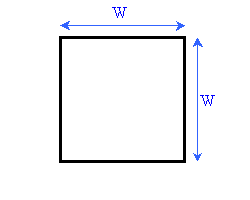Square Loop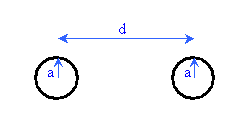Rectangular LoopRound Wire over a Ground Plane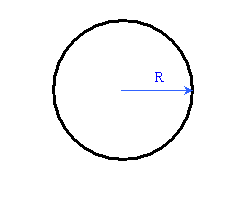Circular LoopTwo Vertically Spaced Traces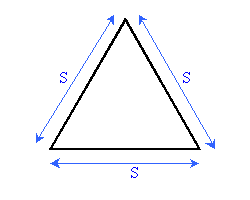Equilateral Triangle Loop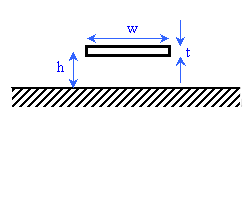Wide Trace over Ground PlaneIsosceles Triangle LoopCoplanar Traces